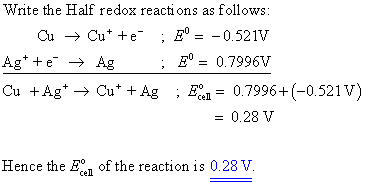# Calculate the E�cell for the following equation. Use these standard potentials

Calculate the E�cell for the following equation. Use these standard potentials .

Cu(s) + Ag+ (aq) —> Cu+ (aq) + Ag(s)